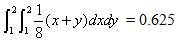# Do The Math Q10

A device runs until either of two components fails, at which point the device stops running.  The joint density function of the lifetimes of the two components, both measured in hours, is

f (x,y)=x+y/8 for 0< x < 2 and 0< y < 2 .

What is the probability that the device fails during its first hour of operation?

1. 0.125
2. 0.141
3. 0.391
Prob. = 1 –